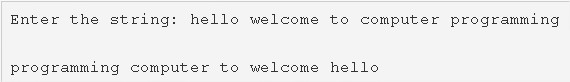# C Program To Reverse Words In A String | C Programs

C program to reverse order of words in a given string – In this article, we will discuss the multiple methods to reverse the order of words in a given string in C programming.

Suitable examples and sample programs have also been added so that you can understand the whole thing very clearly. The compiler has also been added with which you can execute it yourself.

The methods used in the same are as follows:

• Using Standard Method
• Using Function

A string is nothing but an array of characters. The value of a string is determined by the terminating character. Its value is considered to be 0.As given in the example in the uploaded image above, firstly, we need to enter a specific string.

The string uploaded is as follows:

“hello welcome to computer programming”

Thus, a completely reversed string of the same is as follows:

“programming computer to welcome hello”

Hence, the methods to do the same in C programming are as follows:

## Using Standard Method

1. Initialize k=0, j=0.

2) Read the entered string using gets(s). Initialize n=length of the string.

3) Swap the starting index element s[i] with the last index element s[n-i-1].Repeat this step using for loop for(i=0;i<n/2;i++).After all iterations of for loop, we will get reverse ordered string.

4) Insert the location values of the white spaces present in the string, in the array a[] using for loop for(i=0;s[i];i++) , by increasing i,k values.

After for loop initialize a[k] with i value.

5) Reverse the order of letters of each word in the reverse ordered string as

The outer for loop iterates with the structure for(i=0; i<=k;i++)

a) initialize n=a[i]-j.n indicates the length of the word.

b) The inner for loop iterates through the word with  the structure for(l=0;l<n/2;l++)

swap the element with starting index(l+j) with element with ending index (n-l-1) by increasing the l value.

Repeat a,b steps until i<=k.

6) Print the string with reverse order of words.

Output:

### Using Function

1. The swap(char *s1, char *s2) function swaps the character at the pointer variable s1 with the character at the pointer variable s2.

2) The stringlength(char *s) function will return the length of the given string.

3) The main() function calls the reverse(char *s) function, passing the string as an argument to the function. The reverse() function reverses the order of the words in the string.

4) The reverse() function calls the stringlength() function to get the length of the string and initialize the length value to n.

a) Calls the stringlength() function to get the length of the string and initialize the length value to n.

b) For loop iterates, through the string from i=0 to i<n/2.

It calls the swap() function to swap the element at starting index i with the element at last index (n-i-1).

The reverse function calls the swap function repeatedly until i<n/2.After all iterations of for loop, we will get the reverse ordered string.

c) It finds the locations of the white space in the string and stores the location values into the string a[] using for loop for(i=0;s[i];i++) and with increasing i, k values.

d) For loop iterates from i=0 to i<k

Initialize n with the length of the word.i.e a[i]-j.

The reverse function calls the swap function to swap the letter at the starting index (l+j) of the word with the letter at the last index (a[i]-l-1).

Initialize j=a[i]+1.

It calls the swap function repeatedly until i<k.

After all iterations of for loop, we will get the string with reverse ordered words.

5) Print the string with reverse order of words.

Output:

x

## C Program To Print Number Of Days In A Month | Java Tutoring

C program to input the month number and print the number of days in that ...